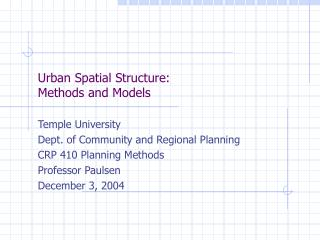DownloadDownload PresentationUrban Spatial Structure: Methods and Models

# Urban Spatial Structure: Methods and Models

Télécharger la présentation## Urban Spatial Structure: Methods and Models

- - - - - - - - - - - - - - - - - - - - - - - - - - - E N D - - - - - - - - - - - - - - - - - - - - - - - - - - -
##### Presentation Transcript

1. Urban Spatial Structure:Methods and Models Temple University Dept. of Community and Regional Planning CRP 410 Planning Methods Professor Paulsen December 3, 2004

2. Today’s lecture • Demonstrate core features of “distance-based” and “spatial-interaction” models, which can be useful in modeling spatial structures of interest to planners.

3. Spatial modeling, generally • Many of the data sets planners utilize, and many of the phenomena we are interested in are inherently spatial. • Our analysis can model spatial processes and relationships explicitly. • Frequently, we essentialize “space” to mean “distance” (and drive real geographers crazy)

4. Spatial modeling • A general class of models is called “spatial interaction” models, which says that the interaction between two places (cities, blocks, regions, etc) is a function of the distance between these two places.

5. Urban Spatial Structure • A common model of urban spatial structure in Urban Economics is the “Monocentric” Alsonso-Mills-Muth model. • In this model, density/intensity of land uses is a function of the distance from CBD (Central Business District)

6. Monocentric (AMM) model Office bid-rent curve Land Value Manufacturing bid-rent curve Residential bid-rent curve d’ d’’ Distance to CBD Density/intensity of use declines with distance Aggregate bid-rent curve is envelope of all bid-rent curves

7. Land Value distance

8. Monocentric (AMM) model • Utilizes a negative exponential distance function: • The population density of a metropolitan region (under certain conditions) follows the relationship: • The density at location i, which is distance di from the center (do), where gamma is the “gradient” of distance (the rate at which density falls from distance to center)

9. Monocentric (AMM) model • The negative exponential density function is empirically estimable using OLS regression by taking a logarithmic transformation.

10. Estimation of AMM model • For spatial units (eg. Census tracts) calculate density and distance from a central point. Estimate regression of form: Independent variable: distance Dependent Variable: ln of density Constant Estimated coefficient on distance

11. Estimation of AMM model • For Philadelphia PMSA census block groups, acquire data on 2000 population and distance from City Center.

12. ln of Population Density Distance from Philadelphia City Center (miles) Non-parametric Kernel density estimation of Monocentric Model for Philadelphia MSA Output created using EasyReg by Herman Bierens

13. Spatial interaction models • Spatial interaction is the flow of goods, people, information, etc. between two places • Most generally, the interaction between two regions i and j is a function of the properties of regions i and j and the “distance” between them. • Relevant properties may include number of jobs, number of destinations, square feet of retail space, population, income …

14. Gravity models • Gravity models are the most commonly known type of Spatial Interaction Model, based on Newtonian physics. Recall from Physics 101 that the gravitational force exerted between two bodies is a of the product of their masses over the distance between them squared, times the gravitational constant k.

15. Gravity models • Likewise, we can hypothesize that the number of job-trips between point A and B is a function of the population at A and the number of jobs at B and the distance between them.

16. Gravity models • Let’s specify the form more generally: • We read this as the interaction T between places i (origin) and j (destination) is a function of a constant k, a measure of the “potential” p at place i and j, over distance raised to some power lambda, where alpha and beta are parameters.

17. Gravity models • Two approaches to “using” gravity models. • 1. Use theory and generally accepted practice to predict flows between i and j, based on knowledge of Pi and Pj • E.g. Based on populations and jobs data, predict commuting flows between TAZi and TAZj • 2. Use data on actual flows to estimate empirically the parameters of a gravity model.

18. Gravity models • Some modeling choices: • Distance decay function. Recall the denominator was specified as: • This is called “distance decay” because the interaction of two places “decays” with increased distance between them

19. Distance Decay • Choice of lambda, the distance decay parameter: • Traditionally, and in keeping with Newton’s law of gravity, researchers have used 2. But there is no clear theoretical reason why. • Next slide shows how different values of lambda lead to faster/slower rates of distance decay • Lambda represents the “friction” of distance • We should expect that lambda would be different for different types of interactions

20. Empirical gravity model example • Using data on actual flows, estimate the parameters of the gravity model. • Example: County to County commuting flows in New Jersey • Data: 2000 Census for County Populations. 2000 County employment figures, BEA-REIS (Bureau of Economic Analysis-Regional Economic Information System). Commuting flows, Census Transportation data. Distance measured county centroid to centroid from TIGER files

21. New Jersey commuting flows • Specify the gravity model to be used • Number of commuters between county i and county j is Tij. • Pi is population in county I (origins) • Ej is measure of employment in county j (destinations)

22. New Jersey commuting flows • To make the equation estimable, we take a logarithmic transformation:

23. Gravity models • We can utilize the relationships identified in the gravity model to develop “accessibility” indices for places, used in many land use allocation models. • For each location i, we want to express Ai, how “accessible” destinations of interest (j’s) are